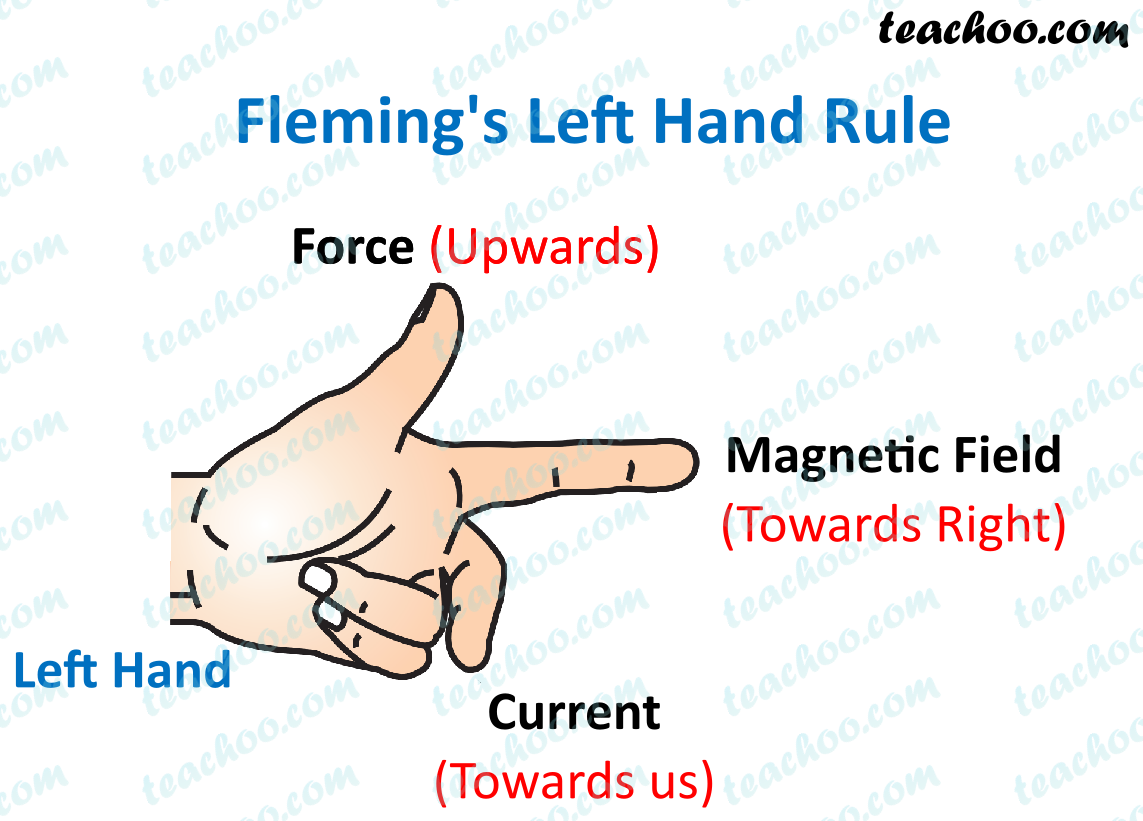Assertion Reasoning questions (MCQ)

Class 10
Chapter 13 Class 10 - Magnetic Effects of Electric Current

## Reason (R): Fleming’s Left hand Rule is applied to measure the induced current.Fleming's left hand rule is used to find the direction of the force/ motion of a conductor.

Fleming's right-hand rule states that if we hold the forefinger, the middle finger and the thumb of our right hand at right angles to one another such that

• Forefinger points in the direction of magnetic field
• Thumb in the direction of the motion of conductor
• Then the direction which the middle finger points gives the direction of the induced current.

Thus,

• Assertion is true.
• Reason is false.

So, the correct answer is (c)

Get live Maths 1-on-1 Classs - Class 6 to 12